• 图像去噪  图像去噪是信号处理的一个经典问题，传统的去噪方法多采用平均或线性方法进行，常用...随着小波理论的日益完善，其以自身良好的时频特性在图像去噪领域受到越来越多的关注，开辟了用非线性方法去噪的先河。
图像去噪
图像去噪是信号处理的一个经典问题，传统的去噪方法多采用平均或线性方法进行，常用的是维纳滤波，但是去噪效果不太好（维纳滤波在图像复原中的作用）。小波去噪
随着小波理论的日益完善，其以自身良好的时频特性在图像去噪领域受到越来越多的关注，开辟了用非线性方法去噪的先河。具体来说，小波能够去噪主要得益于小波变换有如下特点：
（1）低熵性。小波系数的稀疏分布，使图像变换后的熵降低。意思是对信号（即图像）进行分解后，有更多小波基系数趋于0（噪声），而信号主要部分多集中于某些小波基，采用阈值去噪可以更好的保留原始信号。
（2）多分辨率特性。由于采用了多分辨方法，所以可以非常好地刻画信号的非平稳性，如突变和断点等（例如0-1突变是傅里叶变化无法合理表示的），可以在不同分辨率下根据信号和噪声的分布来消除噪声。
（3）去相关性。小波变换可对信号去相关，且噪声在变换后有白化趋势，所以小波域比时域更利于去噪。
（4）基函数选择灵活。小波变换可灵活选择基函数，也可根据信号特点和去噪要求选择多带小波和小波包等（小波包对高频信号再次分解，可提高时频分辨率），对不同场合，选择不同小波基函数。

根据基于小波系数处理方式的不同，常见去噪方法可分为三类：
（1）基于小波变换模极大值去噪（信号与噪声模极大值在小波变换下会呈现不同变化趋势）
（2）基于相邻尺度小波系数相关性去噪（噪声在小波变换的各尺度间无明显相关性，信号则相反）
（3）基于小波变换阈值去噪
小波阈值去噪是一种简单而实用的方法，应用广泛，因此重点介绍。

阈值函数选择
阈值处理函数分为软阈值和硬阈值，设w是小波系数的大小，wλ是施加阈值后小波系数大小，λ为阈值。
（1）硬阈值
当小波系数的绝对值小于给定阈值时，令其为0，而大于阈值时，保持其不变，即：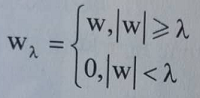（2）软阈值
当小波系数的绝对值小于给定阈值时，令其为0，大于阈值时，令其都减去阈值，即：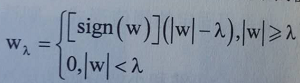如下图，分别是原始信号，硬阈值处理结果，软阈值处理结果。硬阈值函数在|w| = λ处是不连续的，容易造成去噪后图像在奇异点附近出现明显的伪吉布斯现象。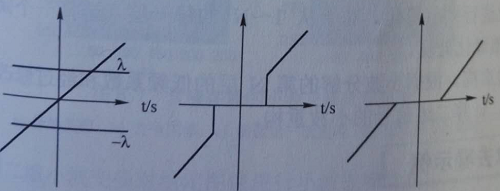阈值大小的选取
阈值的选择是离散小波去噪中最关键的一部。在去噪过程中，小波阈值λ起到了决定性作用：如果阈值太小，则施加阈值后的小波系数将包含过多的噪声分量，达不到去噪的效果；反之，阈值太大，则去除了有用的成分，造成失真。小波阈值估计方法很多，这里暂不介绍。

小波去噪实现步骤
（1）二维信号的小波分解。选择一个小波和小波分解的层次N，然后计算信号s到第N层的分解。
（2）对高频系数进行阈值量化。对于从1~N的每一层，选择一个阈值，并对这一层的高频系数进行软阈值量化处理。
（3）二维小波重构。根据小波分解的第N层的低频系数和经过修改的从第一层到第N的各层高频系数，计算二维信号的小波重构

Matlab函数介绍
（1）wavedec2函数
该函数用于对多尺度二维小波进行分解，其常用调用格式：
[C,S] = wavedec2(X,N,'wname'):用小波函数wname对信号X在尺度N上的二维分解，N是严格正整数。

（2）wrcoef2函数
该函数用于对二维小波系数进行单支重构，其调用格式：
X = wrcoef2('type',C,S,'wname',N):用指定的小波函数wname进行N尺度重构。当type = 'a'时，仅对信号的低频部分进行重构，此时N可以为0;当type = 'h'(或'v'/'d')时，对信号（水平、垂直、对角）的高频进行重构，N为严格正整数。

（3）wthcoef2函数
该函数用于对二维信号的小波系数阈值进行处理，常用调用格式：
NC = wthcoef2('type',C,S,N,T,SORH):返回经过小波分解结构[C,S]进行处理后的新的小波分解向量NC，[NC,S]即构成一个新的小波分解结构。N是一个包含高频尺度的向量，T是相应的阈值，且N和T长度须相等。返回'type'（水平、垂直、对角线）方向的小波分解向量NC。参数SORH用来对阈值方式进行选择，当SORH = 's'时，为软阈值，当SORH =
'h'时，为硬阈值。

小波去噪Matlab实例
clear all;
subplot(2,2,1);image(X);
colormap(map);
xlabel('(a)原始图像');
axis square
%产生含噪声图像
init = 2055615866;
randn('seed',init);
x = X + 50*randn(size(X));
subplot(2,2,2);image(x);
colormap(map);
xlabel('(b)含噪声图像');
axis square
%下面进行图像的去噪处理
%用小波函数coif3对x进行2层小波分解
[c,s] = wavedec2(x,2,'coif3');
%提取小波分解中第一层的低频图像，即实现了低通滤波去噪
%设置尺度向量
n = [1,2];
%设置阈值向量p
p = [10.12,23.28];
%对三个方向高频系数进行阈值处理
nc = wthcoef2('h',c,s,n,p,'s');
nc = wthcoef2('v',nc,s,n,p,'s');
nc = wthcoef2('d',nc,s,n,p,'s');
%对新的小波分解结构[c,s]进行重构
x1 = waverec2(nc,s,'coif3');
subplot(2,2,3);image(x1);
colormap(map);
xlabel('(c)第一次去噪图像');
axis square
%对nc再次进行滤波去噪
xx = wthcoef2('v',nc,s,n,p,'s');
x2 = waverec2(xx,s,'coif3');
subplot(2,2,4);image(x2);
colormap(map);
xlabel('(d)第二次去噪图像');
axis square由于例子简单，处理效果一般，但可以明显地看出高频噪声得到了抑制


展开全文小波变换 图像去噪 MATLAB 图像处理
• 小波指的是一种能量在时域非常集中的波，它的能量有限，都集中在某一点...小波图像处理里被称为图像显微镜，原因在于它的多分辨率分解能力可以将图片信息一层一层分解剥离开来。剥离的手段就是通过低通和高通滤波...
      小波指的是一种能量在时域非常集中的波，它的能量有限，都集中在某一点附近，而且积分的值为零，这说明它与傅里叶波一样是正交波。

图像的傅里叶变换是将图像信号分解为各种不同频率的正弦波。同样，小波变换是将图像信号分解为由原始小波位移和缩放之后的一组小波。小波在图像处理里被称为图像显微镜，原因在于它的多分辨率分解能力可以将图片信息一层一层分解剥离开来。剥离的手段就是通过低通和高通滤波器。

小波变换可以和傅里叶变换结合起来理解。傅里叶变换是用一系列不同频率的正余弦函数去分解原函数，变换后得到是原函数在正余弦不同频率下的系数。小波变换使用一系列的不同尺度的小波去分解原函数，变换后得到的是原函数在不同尺度小波下的系数。不同的小波通过平移与尺度变换分解，平移是为了得到原函数的时间特性，尺度变换是为了得到原函数的频率特性。

小波变换步骤：

1.把小波w(t)和原函数f(t)的开始部分进行比较，计算系数C。系数C表示该部分函数与小波的相似程度。

2.把小波向右移k单位，得到小波w(t-k)，重复1。重复该步骤直至函数f结束.

3.扩展小波w(t)，得到小波w(t/2)，重复步骤1,2.

4.不断扩展小波，重复1,2,3.

haar小波：

我这里使用的haar小波，缩放函数是[1 1]，小波函数是[1 -1]。是最简单的小波了。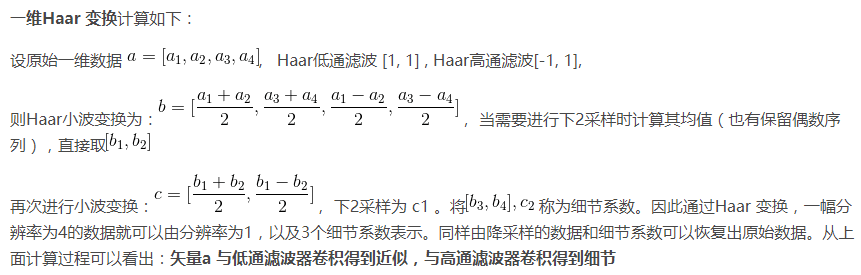图像二维离散小波变换 ：

图像的二维离散小波分解和重构过程如下图所示，分解过程可描述为：首先对图像的每一行进行 1D-DWT，获得原始图像在水平方向上的低频分量 L 和高频分量 H，然后对变换所得数据的每一列进行 1D-DWT，获得原始图像在水平和垂直方向上的低频分量 LL、水平方向上的低频和垂直方向上的高频 LH、水平方向上的高频和垂直方向上的低频 HL 以及水平和垂直方向上的的高频分量 HH。

重构过程可描述为：首先对变换结果的每一列进行以为离散小波逆变换，再对变换所得数据的每一行进行一维离散小波逆变换，即可获得重构图像。由上述过程可以看出，图像的小波分解是一个将信号按照低频和有向高频进行分离的过程，分解过程中还可以根据需要对得到的 LL 分量进行进一步的小波分解，直至达到要求。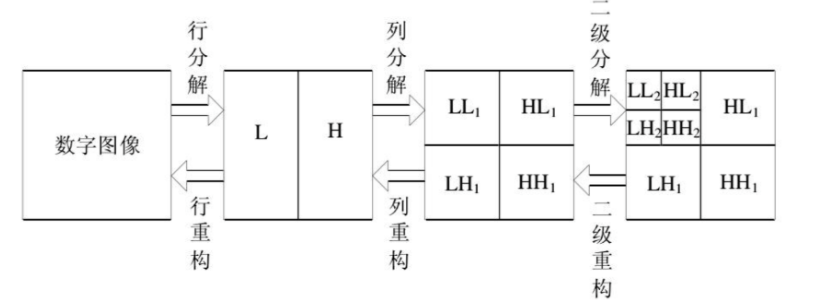对于二维图像Haar变换不再从一个方向进行滤波，而是从水平和竖直两个方向进行低通和高通滤波（水平和竖直先后不影响），用图像表述如图所示：图中a表示原图，图b表示经过一级小波变换的结果，h1 表示水平反向的细节，v1 表示竖直方向的细节，c1表示对角线方向的细节，b表示下2采样的图像。图c中表示继续进行Haar小波变换。一级Haar小波变换实际效果如图3所示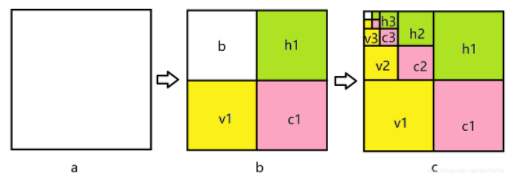matlab实例

小波去噪实现步骤：

（1）二维信号的小波分解。选择一个小波和小波分解的层次N，然后计算信号s到第N层的分解。
（2）对高频系数进行阈值量化。对于从1~N的每一层，选择一个阈值，并对这一层的高频系数进行软阈值量化处理。
（3）二维小波重构。根据小波分解的第N层的低频系数和经过修改的从第一层到第N的各层高频系数，计算二维信号的小波重构

Matlab函数介绍

1、dwt2是二维单尺度小波变换，其可以通过指定小波或者分解滤波器进行二维单尺度小波分解。DWT2的一种语法格式：[cA,cH,cV,cD]=dwt2(X,'wname')；也就是说DWT2只能对某个输入矩阵X进行一次分解。

[cA1, cH1, cV1, cD1] = dwt2(I_noise, 'haar');
figure
subplot(221), imshow(cA1, []);
subplot(222), imshow(cH1, []);
subplot(223), imshow(cV1, []);
subplot(224), imshow(cD1, []);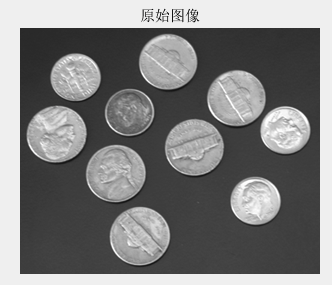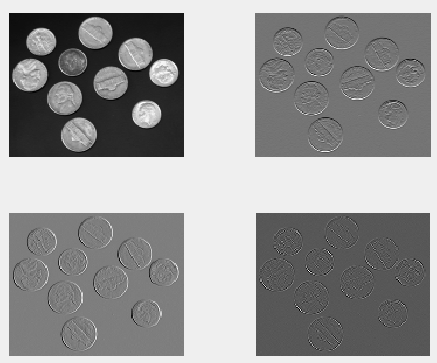可以看出，第一张图是图像的近似，相当于图像的低频部分，而其它三张图是图像的轮廓，也就是水平，垂直和对角三个方向的细节。是图像的高频部分。至此，各变量的维数如下所示。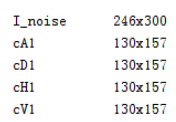2、wavedec2函数

该函数用于对多尺度二维小波进行分解，其常用调用格式：[C,S] = wavedec2(X,N,'wname'):

X：要进行小波分解的图像；
N ：指定分解的层数；
wname：指定用什么小波基进行分解。
输出：
c：为各层分解系数；
s: 各层分解系数长度，也就是大小。
用小波函数wname对信号X在尺度N上的二维分解，其中N为大于1的正整数。可以对输入矩阵X进行N次分解。C代表分解系数的组合，是一个向量：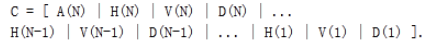C的大小为 [1,img_height×img_width]；A(N)是图像第N层的近似表示，尺度最小，在金字塔中就是每层的下采样的图像，而H、V、D分别表示图像的水平高频分量，垂直高频分量，对角高频分量。正如我们在金字塔概念中所了解的，在第N-1层下采样到N层，N层的图像维度（尺度）是变小了，也就意味着在下采样过程中丢失了信息，而这些丢失的信息实质是高频信息，那么这些信息在小波分解中可以通过HVD这些高频分量来保存。
这里贴上小波分解之后的结果图，直观地感受一下。这里对原始图像进行三层小波分解。红框a表示的就是近似图像。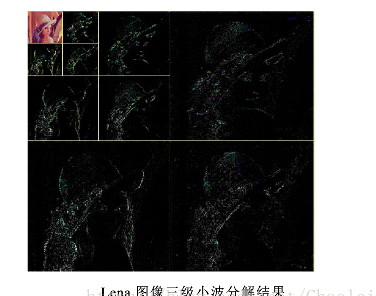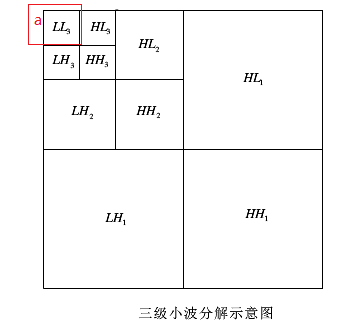需要指出的是，每一次的小波分解都是在近似图像上进行分解。S 是储存各层分解系数长度的,即第一行是A(N)的长度,第二行是H(N)|V(N)|D(N)|的长度,第三行是 H(N-1)|V(N-1)|D(N-1)的长度,倒数第二行是H(1)|V(1)|D(1)长度,最后一行是原始图像img的长度(大小)。 这里原始图像是512×512，并进行了3层的小波分解。对应的s内容如下图：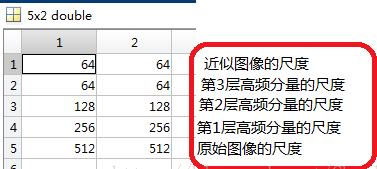S表示每一层分解结果的维数，如果进行n层小波分解，S 的大小是(n+1)*2，最后一行表示的是原始图像的size。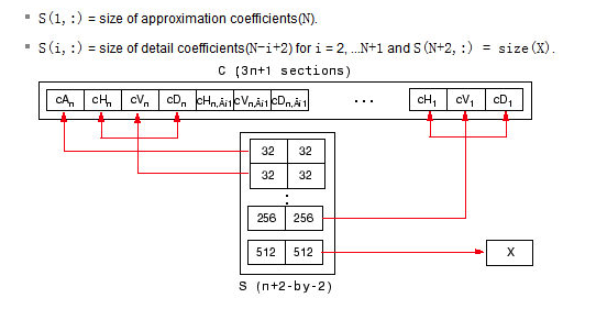3、wdcbm2函数

[thr,nkeep] = wdcbm2(c,s,alpha,m) 返回与level相关的阈值thr和要保持的系数数NKEEP， 函数用于去噪或压缩。使用基于Birge-Massart策略的小波系数选择规则获得thr。通常，alpha= 1.5用于压缩，alpha= 3用于去噪。使用wdcbm2选择各层的独立阈值。

[C，S]是要由wavedec2函数得到的进行去噪或压缩的图像的小波分解结构，level j = size(S,1)-2.

THR是3*j的矩阵，THR（：，j）包含对于level j情况下，水平，对角线和垂直三个方向的阈值。 NKEEP是长度为j的向量，NKEEP（j）包含要保持在级别j情况下系数的数量。

j，M和ALPHA定义策略：

在j + 1级（和更粗略的级别），一切都保留。
对于从1到j的级别i，n_i最大系数保持为n_i = M /（j + 2-i）^ ALPHA。
M的默认值是M = prod（S（1，:)）最粗近似系数的数量。
4、wdencmp函数

[XC,CXC,LXC,PERF0,PERFL2] = wdencmp('lvd',C,S,'wname',N,THR,SORH)
函数wdencmp用于一维或二维信号的消噪或压缩。wname是所用的小波函数，gbl(global的缩写)表示每层都采用同一个阈值进行处理，lvd表示每层用不同的阈值进行处理，N表示小波分解的层数，THR为阈值3*N，SORH表示选择软阈值还是硬阈值(分别取为’s’和’h’)，XC是消噪或压缩后的信号，[CXC，LXC]是XC的小波分解结构，PERF0和PERFL2是恢复和压缩L^2的范数百分比, 是用百分制表明降噪或压缩所保留的能量成分。

5、wrcoef2函数

wrcoef2函数是用来重建一幅图像的系数，其实就是根据小波分解之后的系数c来重建其对应的图像。重建好的图像的尺度与原始图像一致。即无论你要重构哪个层的系数，最终它的维度都是和原始图像的尺度一致。其调用形式如下：
X = wrcoef2(‘type’,c,s,’wname’,N)

type ：指定要进行重构的小波系数，如a –近似图像 ;h – 水平高频分量;v – 垂直高频分量;d–对角高
频分量；
c: 是小波分解函数wavedec2分解的小波系数；
s: 是wavedec2分解形成的尺度；
wname ：指定小波基；
N ：指定重构的小波系数所在的层。 默认重构最大层的系数，N = size(S,1)-2。N所指的层数是如何表示的？比如将图像小波分解成3层，那么N = 3是代表256×256那一层，还是64×64那一层？N=3 代表的是64×64那一层。
wrcoef2 的过程就相当于 appcoef2 或者 detcoef2 （抽取系数）后再进行 upcoef2（重构）。

clear;
close all;

file = 'lena_gray_512.tif';
img = double(img);
% 对图像进行3层的小波分解
N = 3; % 设置分解层数
[c,s] = wavedec2(img,N,'db1');

% 对各层的近似图像a进行重构
a1 = wrcoef2('a',c,s,'db1',1);
a2 = wrcoef2('a',c,s,'db1',2);
a3 = wrcoef2('a',c,s,'db1',3);

6、appcoef2 函数

appcoef2适用于2维图像，其主要是为了提取小波分解中形成的近似图像，即低频分量。
A = appcoef2(c,s,’wname’,N)

c：小波分解的小波系数
s：小波分解的对应尺度
wname ：指定小波基
N ：指定小波系数所在的层数
7、detcoef2

函数detcoef2 用来对二维离散小波变换的高频部分系数进行提取。 其调用形式为：
D = detcoef2(O,c,s,N)

O：指定提取哪个高频分量，取值分别为：’h’ –水平高频 or ‘v’ – 垂直高频 or ‘d’ – 对角高频；
c：小波系数矩阵；
s：尺度矩阵；
8、wthcoef2函数

该函数用于对二维信号的小波系数阈值进行处理，常用调用格式：
NC = wthcoef2('type',C,S,N,T,SORH):返回经过小波分解结构[C,S]进行处理后的新的小波分解向量NC，[NC,S]即构成一个新的小波分解结构。N是一个包含高频尺度的向量，T是相应的阈值，且N和T长度须相等。返回'type'（水平、垂直、对角线）方向的小波分解向量NC。参数SORH用来对阈值方式进行选择，当SORH = 's'时，为软阈值，当SORH = 'h'时，为硬阈值。

9、重构函数 waverec2

waverec2函数是wavedec2的反函数，返回的结果X就是原始图像。其基于小波分解结构[c,s]对矩阵X进行多级小波重构，其中[c,s]是wavedec2函数的返回值。其调用格式如下：
X = waverec2(c,s,’wname’) )

c： 系数矩阵
s： 尺度矩阵
wname ： 指定小波基
值得注意的是，X = waverec2(c,s,’wname’) 相当于 X = appcoef2(c,s,’wname’,0)。

如何进行小波分解：

假设{x1,x2}是一个由两个元素组成的信号，定义这两个元素的平准和细节为：

a = (x1+x2)/2 ；d=(x1-x2)/2

变换实例如下：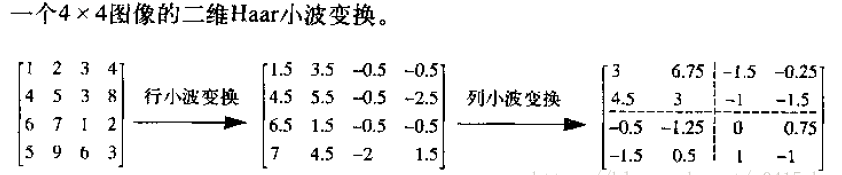from：https://blog.csdn.net/qq_39936376/article/details/80809770

from：http://blog.sina.com.cn/s/blog_84024a4a0101fn02.html

from：https://blog.csdn.net/Chaolei3/article/details/80940459

展开全文• 小波指的是一种能量在时域非常集中的波，它的能量有限，都集中在某一点附近，而且积分的值为零... 小波图像处理里被称为图像显微镜，原因在于它的多分辨率分解能力可以将图片信息一层一层分解剥离开来。剥离的手...
        小波指的是一种能量在时域非常集中的波，它的能量有限，都集中在某一点附近，而且积分的值为零，这说明它与傅里叶波一样是正交波。        图像的傅里叶变换是将图像信号分解为各种不同频率的正弦波。同样，小波变换是将图像信号分解为由原始小波位移和缩放之后的一组小波。        小波在图像处理里被称为图像显微镜，原因在于它的多分辨率分解能力可以将图片信息一层一层分解剥离开来。剥离的手段就是通过低通和高通滤波器。图像二维离散小波变换 ：        图像的二维离散小波分解和重构过程如下图所示，分解过程可描述为：首先对图像的每一行进行 1D-DWT，获得原始图像在水平方向上的低频分量 L 和高频分量 H，然后对变换所得数据的每一列进行 1D-DWT，获得原始图像在水平和垂直方向上的低频分量 LL、水平方向上的低频和垂直方向上的高频 LH、水平方向上的高频和垂直方向上的低频 HL 以及水平和垂直方向上的的高频分量 HH。重构过程可描述为：首先对变换结果的每一列进行以为离散小波逆变换，再对变换所得数据的每一行进行一维离散小波逆变换，即可获得重构图像。由上述过程可以看出，图像的小波分解是一个将信号按照低频和有向高频进行分离的过程，分解过程中还可以根据需要对得到的 LL 分量进行进一步的小波分解，直至达到要求。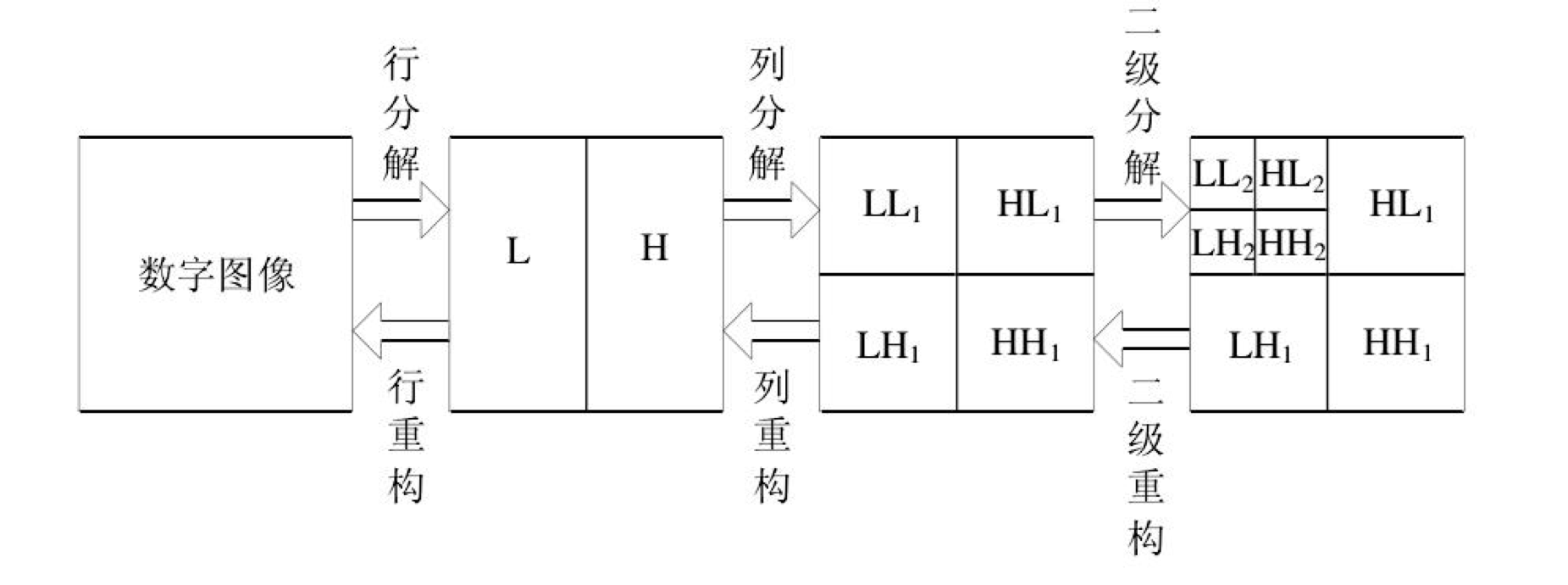图1. 图像二维离散小波变换
展开全文小波变换 图像处理
• 应用小波变换对图像处理 图像金字塔，每一层的像素数为(2^i,2^j):图像金字塔基本操作 对一张图像不断的模糊之后向下采样,得到不同分辨率的图像,同时每次得到的 新的图像宽与高是原来图像的1/2，...
应用小波变换对图像处理
Matlab版本

clear all;
close all;
clc;
tic;
img=img(:,:,1);
img=img(1:512,1:512);
[m n]=size(img);
[LL LH HL HH]=haar_dwt2D(img);
img=[LL LH;HL HH];
imgn=zeros(m,n);
for i=0:m/2:m/2
for j=0:n/2:n/2
[LL LH HL HH]=haar_dwt2D(img(i+1:i+m/2,j+1:j+n/2)); %对一层分解后的四个图像分别再分解
imgn(i+1:i+m/2,j+1:j+n/2)=[LL LH;HL HH];
end
end
toc;

imshow(imgn)

function [LL LH HL HH]=haar_dwt2D(img)
[m n]=size(img);
for i=1:m       %每一行进行分解
[L H]=haar_dwt(img(i,:));
img(i,:)=[L H];
end
for j=1:n       %每一列进行分解
[L H]=haar_dwt(img(:,j));
img(:,j)=[L H];
end
%本来分解不应该加mat2gray的，不过为了有好的显示效果就加上了
LL=mat2gray(img(1:m/2,1:n/2));          %行列都是低频
LH=mat2gray(img(1:m/2,n/2+1:n));        %行低频列高频
HL=mat2gray(img(m/2+1:m,1:n/2));        %行高频列低频  实现图像矩阵的归一化操作
HH=mat2gray(img(m/2+1:m,n/2+1:n));      %行列都是高频

end

function [L H]=haar_dwt(f) %显然，我没有做边界处理，图片最好是2^n*2^n型的
n=length(f);
n=n/2;
L=zeros(1,n);   %低频分量
H=zeros(1,n);   %高频分量
for i=1:n
L(i)=(f(2*i-1)+f(2*i))/sqrt(2);
H(i)=(f(2*i-1)-f(2*i))/sqrt(2);
end

end


OPENCV版本
#include<stdio.h>
#include "cv.h"
#include "cxcore.h"
#include "highgui.h"
// 二维离散小波变换（单通道浮点图像）
void DWT(IplImage *pImage, int nLayer)
{
// 执行条件
if (pImage)
{
if (pImage->nChannels == 1 && pImage->depth == IPL_DEPTH_32F && ((pImage->width >> nLayer) << nLayer) == pImage->width && ((pImage->height >> nLayer) << nLayer) == pImage->height)
//图片为通道，单精度浮点数
{
int i, x, y, n;
float fValue = 0;
int nWidth = pImage->width;
int nHeight  = pImage->height;
int nHalfW = nWidth / 2;
int nHalfH = nHeight / 2;
float **pData  = new float*[pImage->height];
float  *pRow = new float[pImage->width];
float  *pColumn  = new float[pImage->height];
for (i = 0; i < pImage->height; i++)
{
pData[i] = (float*) (pImage->imageData + pImage->widthStep * i);
}
// 多层小波变换
for (n = 0; n < nLayer; n++, nWidth /= 2, nHeight /= 2, nHalfW /= 2, nHalfH /= 2)
{
// 水平变换
for (y = 0; y < nHeight; y++)
{
// 奇偶分离
memcpy(pRow, pData[y], sizeof(float) * nWidth);
for (i = 0; i < nHalfW; i++)
{
x = i * 2;
pData[y][i] = pRow[x];
pData[y][nHalfW + i] = pRow[x + 1];
}
// 提升小波变换
for (i = 0; i < nHalfW - 1; i++)
{
fValue = (pData[y][i] + pData[y][i + 1]) / 2;
pData[y][nHalfW + i] -= fValue;
}
fValue = (pData[y][nHalfW - 1] + pData[y][nHalfW - 2]) / 2;
pData[y][nWidth - 1] -= fValue;
fValue = (pData[y][nHalfW] + pData[y][nHalfW + 1]) / 4;
pData[y] += fValue;
for (i = 1; i < nHalfW; i++)
{
fValue = (pData[y][nHalfW + i] + pData[y][nHalfW + i - 1]) / 4;
pData[y][i] += fValue;
}
// 频带系数
for (i = 0; i < nHalfW; i++)
{
}
}
// 垂直变换
for (x = 0; x < nWidth; x++)
{
// 奇偶分离
for (i = 0; i < nHalfH; i++)
{
y = i * 2;
pColumn[i] = pData[y][x];
pColumn[nHalfH + i] = pData[y + 1][x];
}
for (i = 0; i < nHeight; i++)
{
pData[i][x] = pColumn[i];
}
// 提升小波变换
for (i = 0; i < nHalfH - 1; i++)
{
fValue = (pData[i][x] + pData[i + 1][x]) / 2;
pData[nHalfH + i][x] -= fValue;
}
fValue = (pData[nHalfH - 1][x] + pData[nHalfH - 2][x]) / 2;
pData[nHeight - 1][x] -= fValue;
fValue = (pData[nHalfH][x] + pData[nHalfH + 1][x]) / 4;
pData[x] += fValue;
for (i = 1; i < nHalfH; i++)
{
fValue = (pData[nHalfH + i][x] + pData[nHalfH + i - 1][x]) / 4;
pData[i][x] += fValue;
}
// 频带系数
for (i = 0; i < nHalfH; i++)
{
}
}
}
delete[] pData;
delete[] pRow;
delete[] pColumn;
}
}
}

// 二维离散小波恢复（单通道浮点图像）
void IDWT(IplImage *pImage, int nLayer)
{
// 执行条件
if (pImage)
{
if (pImage->nChannels == 1 && pImage->depth == IPL_DEPTH_32F &&((pImage->width >> nLayer) << nLayer) == pImage->width && ((pImage->height >> nLayer) << nLayer) == pImage->height)
{
int i, x, y, n;
float fValue = 0;
int nWidth = pImage->width >> (nLayer - 1);
int nHeight  = pImage->height >> (nLayer - 1);
int nHalfW = nWidth / 2;
int nHalfH = nHeight / 2;
float **pData  = new float*[pImage->height];
float *pRow = new float[pImage->width];
float *pColumn = new float[pImage->height];
for (i = 0; i < pImage->height; i++)
{
pData[i] = (float*) (pImage->imageData + pImage->widthStep * i);
}
// 多层小波恢复
for (n = 0; n < nLayer; n++, nWidth *= 2, nHeight *= 2, nHalfW *= 2, nHalfH *= 2)
{
// 垂直恢复
for (x = 0; x < nWidth; x++)
{
// 频带系数
for (i = 0; i < nHalfH; i++)
{
}
// 提升小波恢复
fValue = (pData[nHalfH][x] + pData[nHalfH + 1][x]) / 4;
pData[x] -= fValue;
for (i = 1; i < nHalfH; i++)
{
fValue = (pData[nHalfH + i][x] + pData[nHalfH + i - 1][x]) / 4;
pData[i][x] -= fValue;
}
for (i = 0; i < nHalfH - 1; i++)
{
fValue = (pData[i][x] + pData[i + 1][x]) / 2;
pData[nHalfH + i][x] += fValue;
}
fValue = (pData[nHalfH - 1][x] + pData[nHalfH - 2][x]) / 2;
pData[nHeight - 1][x] += fValue;
// 奇偶合并
for (i = 0; i < nHalfH; i++)
{
y = i * 2;
pColumn[y] = pData[i][x];
pColumn[y + 1] = pData[nHalfH + i][x];
}
for (i = 0; i < nHeight; i++)
{
pData[i][x] = pColumn[i];
}
}
// 水平恢复
for (y = 0; y < nHeight; y++)
{
// 频带系数
for (i = 0; i < nHalfW; i++)
{
}
// 提升小波恢复
fValue = (pData[y][nHalfW] + pData[y][nHalfW + 1]) / 4;
pData[y] -= fValue;
for (i = 1; i < nHalfW; i++)
{
fValue = (pData[y][nHalfW + i] + pData[y][nHalfW + i - 1]) / 4;
pData[y][i] -= fValue;
}
for (i = 0; i < nHalfW - 1; i++)
{
fValue = (pData[y][i] + pData[y][i + 1]) / 2;
pData[y][nHalfW + i] += fValue;
}
fValue = (pData[y][nHalfW - 1] + pData[y][nHalfW - 2]) / 2;
pData[y][nWidth - 1] += fValue;
// 奇偶合并
for (i = 0; i < nHalfW; i++)
{
x = i * 2;
pRow[x] = pData[y][i];
pRow[x + 1] = pData[y][nHalfW + i];
}
memcpy(pData[y], pRow, sizeof(float) * nWidth);
}
}
delete[] pData;
delete[] pRow;
delete[] pColumn;
}
}
}

int main( int argc, char** argv )
{
// 小波变换层数
int nLayer = 1;
// 输入彩色图像
// 计算小波图象大小
CvSize size = cvGetSize(pSrc);
if ((pSrc->width >> nLayer) << nLayer != pSrc->width)
{
size.width = ((pSrc->width >> nLayer) + 1) << nLayer;
}
if ((pSrc->height >> nLayer) << nLayer != pSrc->height)
{
size.height = ((pSrc->height >> nLayer) + 1) << nLayer;
}
// 创建小波图象
IplImage *pWavelet = cvCreateImage(size, IPL_DEPTH_32F, pSrc->nChannels);
if (pWavelet)
{
// 小波图象赋值
cvSetImageROI(pWavelet, cvRect(0, 0, pSrc->width, pSrc->height));
cvConvertScale(pSrc, pWavelet, 1, -128);
cvResetImageROI(pWavelet);
// 彩色图像小波变换
IplImage *pImage = cvCreateImage(cvGetSize(pWavelet), IPL_DEPTH_32F, 1);
if (pImage)
{
for (int i = 1; i <= pWavelet->nChannels; i++)
{
cvSetImageCOI(pWavelet, i);
cvCopy(pWavelet, pImage, NULL);
// 二维离散小波变换
DWT(pImage, nLayer);
// 二维离散小波恢复
// IDWT(pImage, nLayer);
cvCopy(pImage, pWavelet, NULL);
}
cvSetImageCOI(pWavelet, 0);
cvReleaseImage(&pImage);
}
// 小波变换图象
cvSetImageROI(pWavelet, cvRect(0, 0, pSrc->width, pSrc->height));
cvConvertScale(pWavelet, pSrc, 1, 128);
cvResetImageROI(pWavelet); // 本行代码有点多余，但有利用养成良好的编程习惯
cvReleaseImage(&pWavelet);
}
// 显示图像pSrc

cvNamedWindow("pSrc", 1);
cvShowImage( "pSrc", pSrc);
cvWaitKey(0); //等待按键
cvDestroyWindow( "pSrc" );
cvReleaseImage(&pSrc);
return 0;
}
展开全文数字图像处理 小波变换 matlab
• 原文地址：小波变换在图像处理中的应用作者：茉莉清茶小波变换在图像处理中的应用 一 小波与图像去噪   图像在采集，转换和传输过程中常常受到成像设备和外部环境噪声干扰等影响产生噪声。小波去噪是利用小波变换中...

原文地址：小波变换在图像处理中的应用作者：茉莉清茶小波变换在图像处理中的应用
一 小波与图像去噪

图像在采集，转换和传输过程中常常受到成像设备和外部环境噪声干扰等影响产生噪声。小波去噪是利用小波变换中的变尺度特性对确定信号具有一种“集中”的能力，当图像信号的能量集中于少数小波系数上，那么这些系数的值必定大于能量分散的大量噪声小波系数值。只要选取适当的阈值，舍去绝对值小于阈值的小波系数，就可实现图像的降噪。
二 小波与图像压缩

小波变换最成功的图像处理应用领域之一就是图像压缩。小波变换将强相关的空间像素阵影射成完全不相关的，能量分布紧凑的小波系数阵，占少数的大的小波系数代表了图像中最主要的能量成分，占多数的小的小波系数表示了一些不重要的细节分量，通过量化去除小系数多代表的细节分量，用很少的码子来描述大系数所代表的主要能量成分，从而达到高的压缩比。小波图像压缩的研究表明，现代应用所需要的许多特征如多分辨，多层质量控制，嵌入式码流等与小波图像编码结构非常自然地融合在一起，在较大压缩比下，小波图像压缩重构质量明显优于DCT变换的方法。因此，在新一代静止图像压缩标准JPEC2000中，采用小波图像编码作为核心算法。
三 小波与图像分割

图像分割是计算机视觉和图像理解的最基本问题，分割结果决定图像处理系统高层模块的性能。图像分割按照一定准则把图像划分为若干互不相交的区域，别分割的区域应满足同质性和唯一性。

由于边缘上图像的灰度级不连续，具有奇异性。基于小波变换的边缘检测进行图像分割，即利用小波系数的多分辨率特征，利用小波分析的局部化特性，获得不同尺度下领域特征。根据这些小波特征可以进行模式分类，图像分割。另外，利用小波分解后的高频信息，还可以进行多尺度边缘检测。

目前，基于小波分析的图像分割与边缘检测可以分为两大类：一类是基于滤波器尺度的多尺度图像分割方法；或是直接构造边缘算子作用于原图像函数以检测边缘；或是先通过小波变换获得图像地多尺度特征，然后对像素进行分类和分割。另一种是构造基于像素点外的尺寸及灰度级差的多尺度函数，并以此函数构造边缘映射。这种方法集成了边界和区域处的特征信息，具有潜在的研究价值。
四 小波变换在图像融合中的应用

这里以两幅图像的融合为例,多幅图像的融合方法可依此类推。基于小波变换的融合算法的流程阐述如下:首先,对已配准的源图像进行小波分解,相当于使用一组高低通滤波器进行滤波,分离出高频信息和低频信息;其次,对每层分解得到的高频信息和低频信息依据所得到的信息特点,采取不同的融合策略,在各自的变换域进行特征信息抽取,分别进行融合;最后,采用第一步的小波变换的重构算法对处理后的小波系数进行反变换重建图像,即可得到融合图像。
Mallat 提出了小波变换的分解与重构的快速算法。对一维信号f(x),分解公式为：

为分辨率 下的尺度函数， 为分辨率 下的小波函数。 、 分别为逼近系数和细节系数。

小波变换利用Mallat提出的小波多分辨率分析思想及小波的分解和重构快速算法进行图像融合。用小波变换可以将图像分解为更低分辨率水平上的低频轮廓信息和原始信号在水平、垂直和对角线方向的高频细节信息,且可以对图像作多次分解,形成多级子带信号。小波运算往往通过小波分解后小波系数的替换、选择、权值或叠加运算进行融合,运算的规则由小波系数的大小、统计值或基于小波变换特性定义的新判决量来确定。从目前融合效果上看,小波变换是一种复杂但融合效果较好的算法,也是一种很有前途的算法。

对待融合图像进行多层小波分解，得吐血的低频分量和图像的高频分量，然后分别对低频分量和高频分量采取相应的任何算子和融合规则进行融合处理，得到融合图像的低频分量和高频分量，然后进行小波逆运算得到融合后的图像，融合原理图如下图所示：

图像A

小波变换系数A

融合后 图  像

融   合

规   则

小波变换系数B

图像B

融合后小波系数

图1 小波变换图像融合流程示意图

该系统的执行步骤如下:
(1)
根据Mallat 算法对原始图像进行小波分解, 得到其小波变换系数。
(2)
由原始图像的低频图像根据一定的融合准则, 确定融合图像的逼近图像。
(3)
对高频系数应用Cross- band- window算法, 确定融合图像的高频系数。
(4)
对融合后的系数进行逆小波变换, 获得融合后的图像。
由于变换后的低频系数和高频系数分别表示不同的图像特征, 因此, 一般采用不同的融合方法。低频系数表示图像的近似信息,
一般求初始图像的平均, 或者把初始图像之一的低频系数直接作为融合图像的低频分量。
例1  基于小波变换图像融合程序及结果分析
subplot(1,3,1), imshow(imgMul), xlabel ('(a)高分辨率多光谱影像');
subplot(1,3,2), imshow(imgPan), xlabel ('(b)多光谱影像');
mulR = imgMul (:,:,1);
mulG = imgMul (:,:,2);
mulB = imgMul (:,:,3);
[Cpan,Lpan] = wavedec2(imgPan,3,'db13');
imgWH = Lpan(1,:);
length = imgWH(1)*imgWH(2);
[Ctmr,Ltmr] = wavedec2(imgR,3,' db13' );
Cr = Cpan; Cr(1:length) = Ctmr(1:length);
[Ctmg,Ltmg] = wavedec2(imgG,3,' db13' );
Cg = Cpan; Cg(1:length) = Ctmg(1:length);
[Ctmb,Ltmb] = wavedec2(imgB,3,' db13' );
Cb = Cpan; Cb(1:length) = Ctmb(1:length);
imgResult(:,:,1) = waverec2(Cr,Lpan,' db13' );
imgResult(:,:,2) = waverec2(Cg,Lpan,' db13' );
imgResult(:,:,3) = waverec2(Cb,Lpan,' db13' );
imwrite(uint8(imgResult), ' Merge.bmp' );
subplot(1,3,3), imshow(uint8(imgResult), xlabel (' (c)小波融合图像'
);

图1 高分辨率影像

图2 多光谱影像

图3 小波变换后影像
与传统的图像融合方法如HIS等相比, 小波融合模型不仅能够针对输入图像的不同特征来合理选择小波变换的次数,
而且在融合操作时又可以根据实际需要来引入双方的细节信息, 从而表现出更强的针对性和实用性, 融合效果更好。另外,
从实施过程的灵活性方面评价, HIS 变换只能而且必须同时对三个波段进行融合操作,
小波方法能够完成对单一波段或多个波段的融合运算。
例2  基于小波变换图像复原程序结果及分析
%下面装入原始图像，X中含有被装载的图像
%画出原始图像
subplot(221);image(X);colormap(map);
title('原始图像');
axis square
%产生含噪声图像
init=2055615866;randn('seed',init)
x=X+10*randn(size(X));
%画出含噪声图像
subplot(222);image(X);colormap(map);
title('含噪声图像');
axis square
%下面进行图像的去噪处理
%用小波画数coif3对x进行2层小波分解
[c,s]=wavedec2(x,2,'coif3');
%提取小波分解中第一层的低频图像，即实现了低通滤波去噪
%设置尺度向量n
n=[1,2]
p=[10.12,23.28];
%对三个方向高频系数进行阈值处理
nc=wthcoef2('h',c,s,n,p,'s');
nc=wthcoef2('v',c,s,n,p,'s');
nc=wthcoef2('d',c,s,n,p,'s');
%对新的小波分解结构[nc，s]进行重构
xx=waverec2(nc,s,'coif3');
%画出重构后图像的波形
subplot(223);image(X);colormap(map);
title('去噪后的图像');
axis square；

原始图像
含噪声图像
去噪后图像

图4 去噪例三

二维信号在应用中一般表现为图像信号，二维信号在小波域中的降噪方法的基本思想与一维情况一样，在阈值选择上，可以使用统一的全局阈值，有可以分作三个方向，分别是水平方向、竖直方向和对角方向，这样就可以把在所有方向的噪声分离出来，通过作用阈值抑制其成分。


展开全文•  根据小波的多分辨率分析原理将图像进行多级二维离散小波变换，可以将图像分解成图像近似信号的低频子带和图像细节信号的高频子带。其中，图像中大部分的噪声和一些边缘细节都属于高频子带，而低频图像处理 机器学习 小波变换 图像增强
• 内容完全转载： 小波理论的基本概念及概述（第二版） 欢迎阅读此份关于小波变换的入门教程。小波变换是一个相对较新的概念（其出现大约是在20世纪...换言之，大多数介绍小波变换的文献对那些小波新手们来说用处不图像处理 小波变换
• 4.Experiment Steps Result and Conlusion：1、了解各种小波性质2、观察各种小波函数及其尺度函数3、获得小波滤波器4、二维小波变换函数与重构函数5、查看及处理小波系数6、用小波变换进行图像压缩与边缘提取【附录...计算机视觉
• 小波变换在数字图像处理中的应用是小波变换典型的应用之一。由信号分析中傅里叶变换的不足引出小波变 换, 然后简单介绍了小波变换的定义和种类, 分析了小波变换的性质和 Mallat 算法, 总结了小波变换在数字图像处理...
• 第十二章 小波变换 目录 1 引言 2 连续小波变换 3 二进小波变换 3.1 Haar变换 4 离散小波变换 4.1 多分辨率分析 4.2 快速小波变换算法 4.3 离散小波变换的设计 4.4 二维离散小波变换 4.5 双...图像处理 数字图像 小波变换 transform
• 这里举一个haar小波作为实例： 假设{x1,x2}是一个由两个元素组成的信号，定义这两个元素的平准和细节为： a = (x1+x2)/2 d=(x1-x2)/2 变换实例如下：  以matlab模拟一下结果如下： 显示原始图像： ...
• 小波变换是多尺度多分辨率的分解方式，可以将噪声...小波图像去噪处理 clear; [A,map]=imread('lenna.jpg'); X=rgb2gray(A); %画出原始图像 subplot(2,2,1);imshow(X); title('原始图像'); %产生含噪图像 x...
• 最近在看物体识别论文摘要，好多论文中涉及到使用离散余弦傅里叶变换DFT（Discrete Fourier Transform）对图像进行处理，因此特地看了这部分的内容，傅里叶变换和小波变换。一、DFT的原理：以二维图像为例，归一化的...离散傅里叶变换 小波变换
• 摘要 本文从二维小波理论出发，对其在图像处理的应用上进行了一些分析和处理，力图反映出小波分析在图像处理方面有着其独特的特点。本文就以下几点进行阐述：①小波基本概念 ②图像压缩 ③图像消噪 ④图象增强 ⑤...图像处理 image c 算法
• 小波变换（wavelet transform，WT）是一种新的变换分析方法，它继承和发展了短时傅立叶变换局部化的思想，同时又克服了窗口大小不随频率变化等缺点，能够提供一个随频率改变的“时间-频率”窗口，是进行信号时频分析...MATLAB 小波变换
• 1.前言： 图像融合是信息融合的一个重要分支，广泛地用于目标识别、机器视觉、智能系统、医学图像处理等领域。传统图像融合方法方法主要是在时间域通过算术运算实现融合，具有算法简单...2.小波图像融合的观点：
• 数字图像处理 第7章 小波和多分辨率处理 小波性质 1、可分离性（xyz）、可伸缩性、平移性 2、多分辨率的兼容性 3、正交性   FWT(二维快速小波变换) 频率耦合滤波器 小波族wfamily的描述信息：...
• 小波分析法开辟了非线性降噪的先河，小波能够降噪得益于小波变换的以下特点：低熵性（小波系数稀松分布，使图像变换后的熵降低）、多分辨率特性（极好的刻画了信号的非平稳性）、去相关性（噪声在变换后有白化趋势，...
• 图像和噪声在经过小波变换后具有不同的特性，因为将含噪信号在各尺度上进行小波分解后，图像的能量主要集中在低分辨率子带上，而噪声信号的能量主要分布在各个高频子带上。 原始图像信息的小波系数绝对值较大，...
...